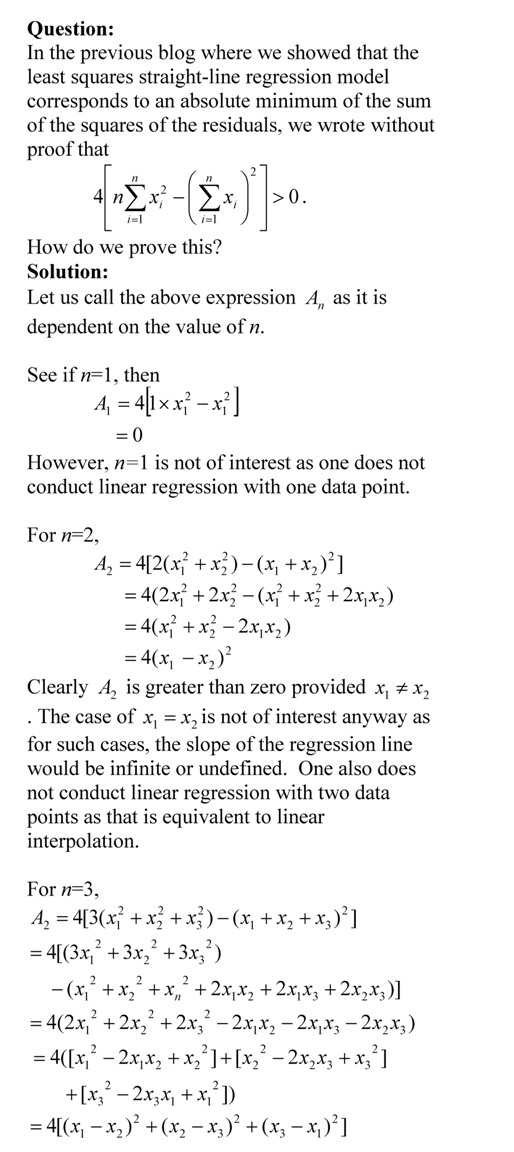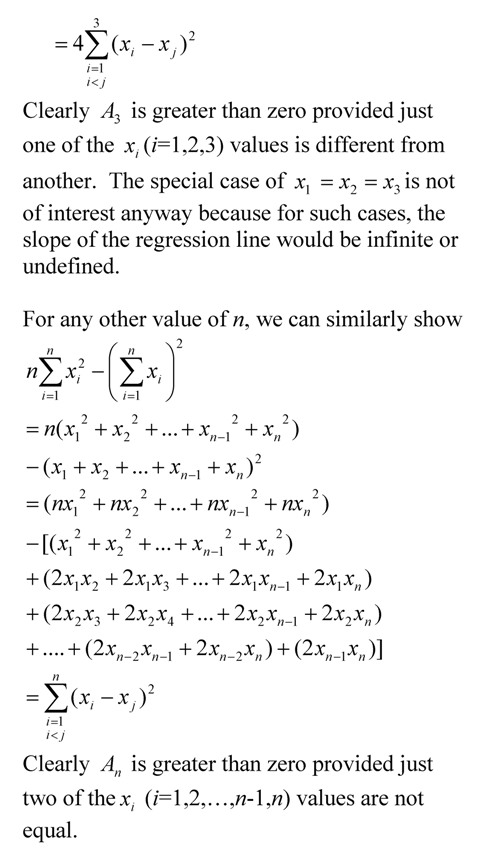## Proving the denominator of the linear regression formula for its constants is greater than zero.

In a previous blog, we wrote without proof that the denominator of the constants of the linear regression formulas are greater than zero.  In this blog, we show the proof.This post is brought to you by Holistic Numerical Methods: Numerical Methods for the STEM undergraduate at http://nm.mathforcollege.com, the textbook on Numerical Methods with Applications available from the lulu storefront, the textbook on Introduction to Programming Concepts Using MATLAB, and the YouTube video lectures available athttp://nm.mathforcollege.com/videos.  Subscribe to the blog via areader or email to stay updated with this blog. Let the information follow you.